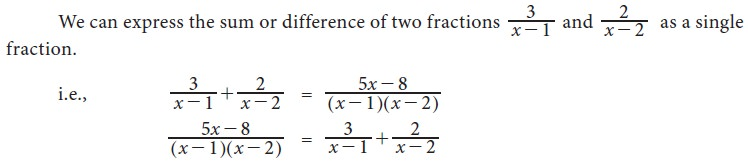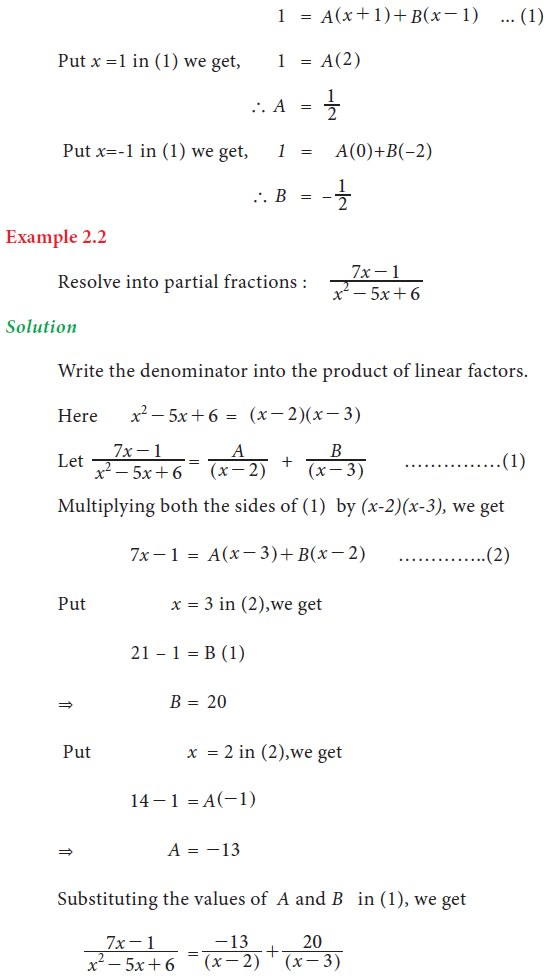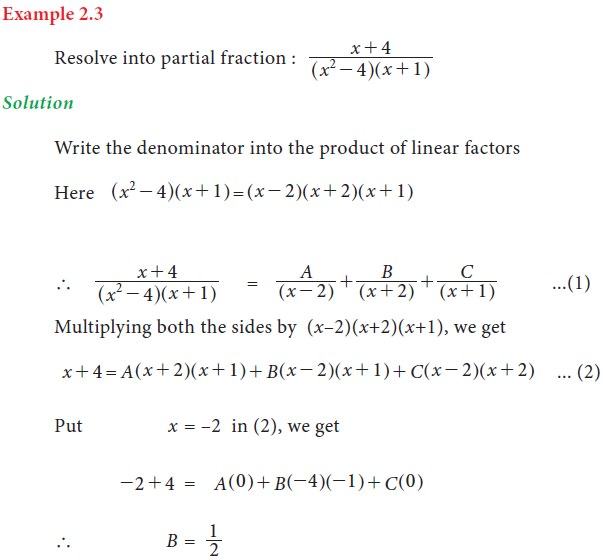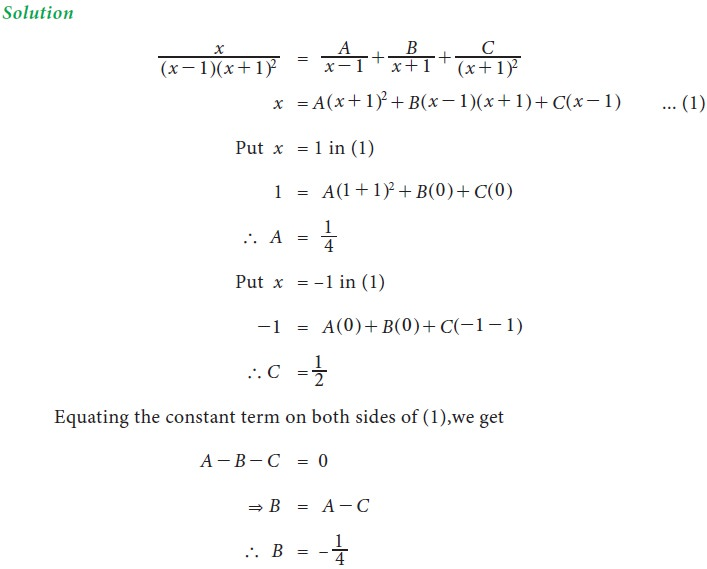Home | | Business Maths 11th std | Partial Fractions

# Partial Fractions

Algebra is a major component of mathematics that is used to unify mathematics concepts.

Introduction

Algebra is a major component of mathematics that is used to unify mathematics concepts. Algebra is built on experiences with numbers and operations along with geometry and data analysis. The word “algebra” is derived from the Arabic word “al-Jabr”. The Arabic mathematician Al-Khwarizmi has traditionally been known as the “Father of algebra”. Algebra is used to find perimeter, area, volume of any plane figure and solid.Partial Fractions

Rational ExpressionAn improper fraction can be expressed as the sum of an integral function and a proper fraction.Partial FractionsProcess of writing a single fraction as a sum or difference of two or more simpler fractions is called splitting up into partial fractions.

Generally if p (x)  and q (x)  are two rational integral algebraic functions of x and the fraction  p(x)/q(x) be expressed as the algebraic sum (or difference) of simpler fractions according to certain specified rules, then the fraction p(x)/q(x) is said to be resolved into partial fractions.

## 1. Denominator contains non-repeated Linear factors## 2. Denominator contains Linear factors, repeated n times## 3. Denominator contains quadratic factor, which cannot be factorized into linear factorsTags : Introduction, Definition, Formula, Solved Example Problems, Exercise | Mathematics , 11th Business Mathematics and Statistics(EMS) : Chapter 2 : Algebra
Study Material, Lecturing Notes, Assignment, Reference, Wiki description explanation, brief detail
11th Business Mathematics and Statistics(EMS) : Chapter 2 : Algebra : Partial Fractions | Introduction, Definition, Formula, Solved Example Problems, Exercise | Mathematics